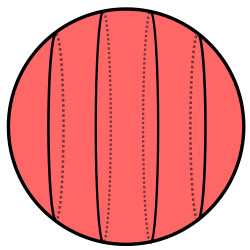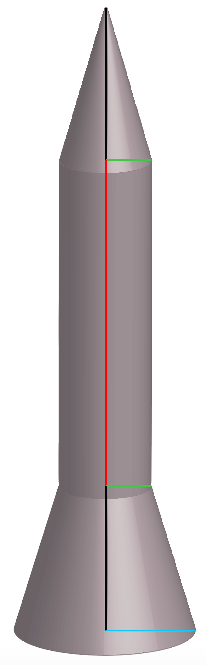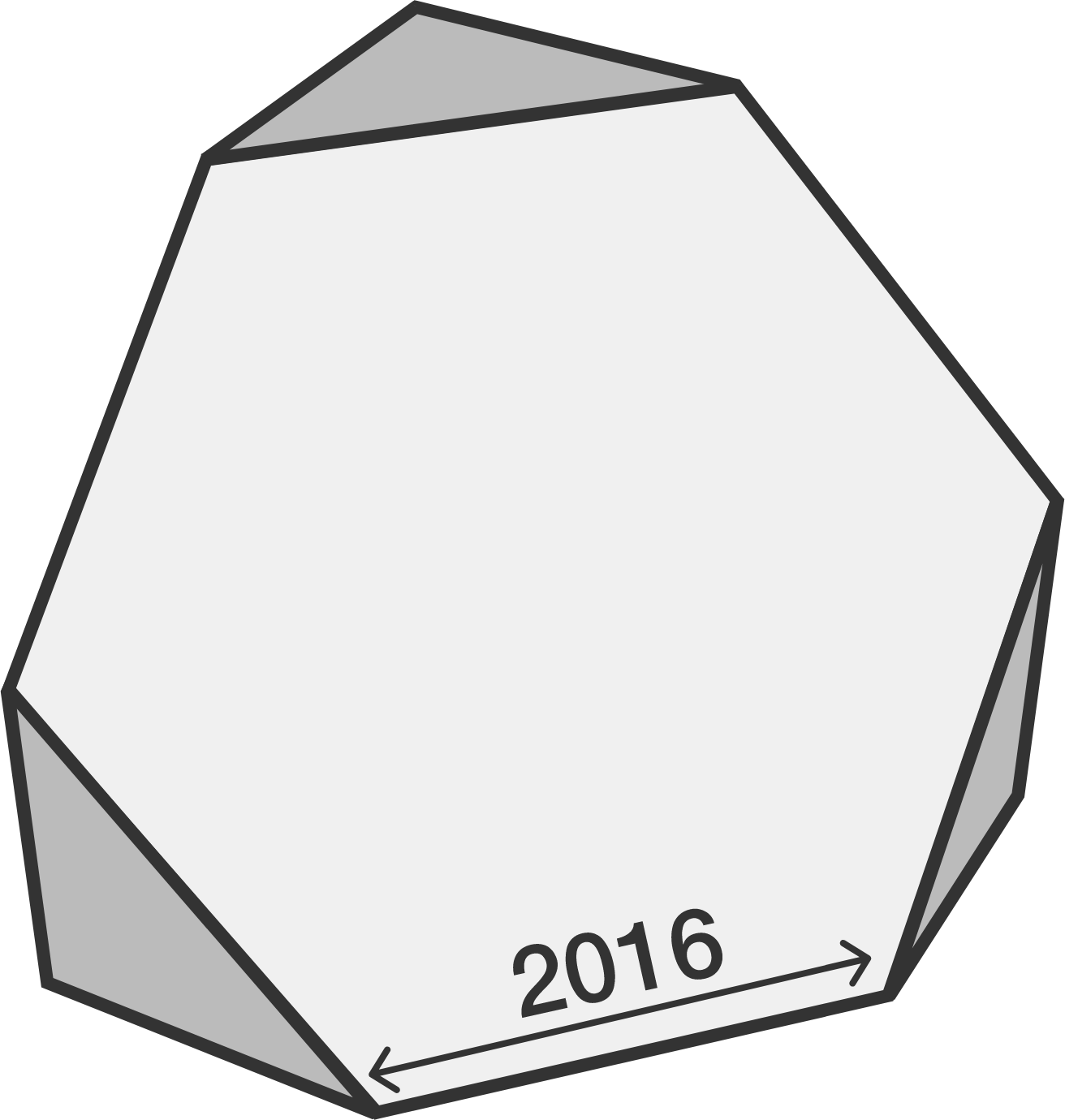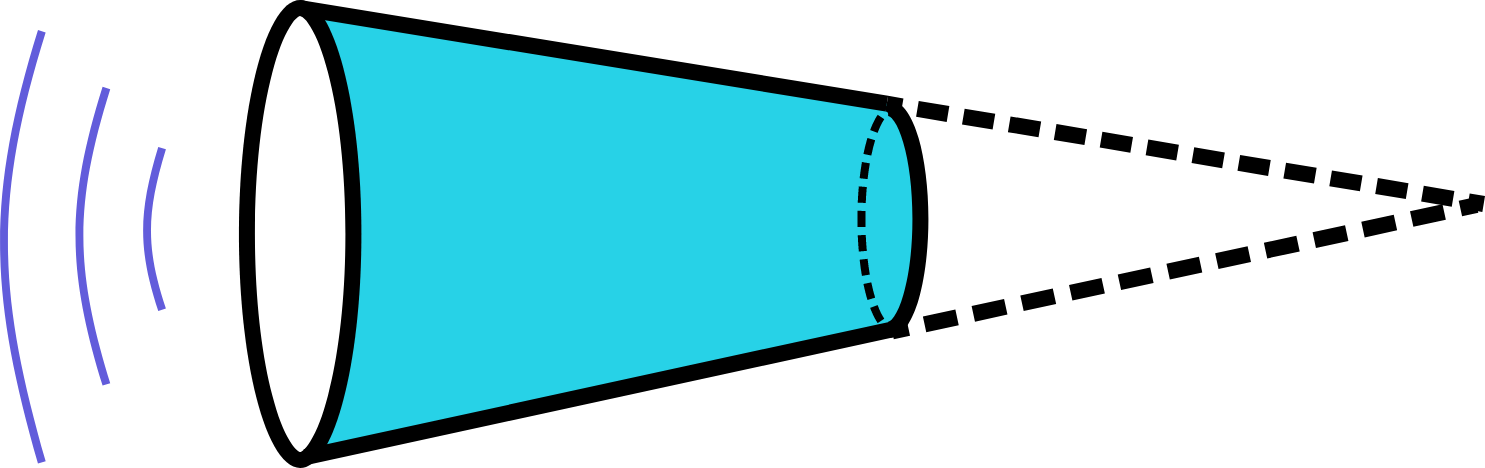Geometry

# Surface Area: Level 3 ChallengesWhen 88 cubes are rearranged to form the cube-sphere in the diagram above, their total surface area decreases by 384.

Find the total surface area of the cube-sphere.

Note: The cube-sphere is solid.

Beginning with a sphere of radius 1, what is the minimum amount of planar 'slices' through the sphere so that the total surface area of the resultant pieces is greater than 50? Here's one potential method:If the picture is too small, right click/two-finger click it and open it in a new tab.

In this above rocket picture,

• The top black line serves as the height of the cone portion of the rocket and the bottom black line serves as the height of the "lamp shade" portion of the rocket. Both black lines have a length of 5 inches.
• The red line serves as the height of the cylindrical portion of the rocket and has a length of 10 inches.
• The green line serves as the radius of the cone portion and cylindrical portion. The green line also serves as the smaller radius of the lampshade portion. The length of the green line is 1 inch.
• The blue line serves as the larger radius of the lampshade portion, and has a length of 2 inches.

If the surface area of the rocket (in square inches) equals $SA$, find $\left \lfloor SA \right \rfloor$.The diagram to the right shows a cube sliced in half.

Find the total surface area of the sliced cube to the nearest integer.You're making a megaphone by wrapping a piece of paper up as a simple cone and then cutting it at half of its height. The 2 new circular bases are parallel and left open at both ends for the air to flow through.

If this megaphone has a height of 24 cm. with a radius of 14 cm. at its bigger base, find its lateral surface area in $\text{cm}^2$.

If your answer is in a form of $\pi\times A$, then submit $A$ as your answer.

×